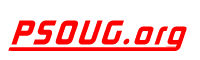Quick Search: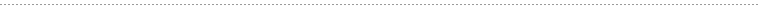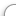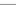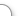CODE Oracle PL/SQL Code Library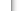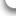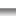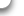JOBS Find Or Post Oracle JobsFORUM Oracle Discussion & ChatThe Oracle PL/SQL REGR_R2 Function      [Return To Index] Jump to: Select a Location PSOUG Home Page Oracle Code Library mySQL Code Library PHP Code Library JavaScript Code Library Oracle Terms & Definitions Oracle Error Codes PSOUG Community Blogs Oracle Jobs Board PSOUG Forum Oracle User Group Directory Free Oracle Magazines Online Learning Center PSOUG Presentations Advanced Code Search News and Events Sponsors Page Submit Code Contact UsLooking for the original pages? (formerly called "Morgan's Library") You can find them here.# Term: REGR_R2

Definition:
In Oracle PL/SQL, the function REGR_R2 is a linear regression analytic function which takes two numeric inputs and returns a numeric output which denotes how well a regression line is fitted. Another way of stating this is that it returns the coefficient of determination, or R-squared, of the regression line. Its return value is determined based on the conditions shown below:

NULL, if VAR_POP (y) = 0
1, if VAR_POP(x) = 0 and VAR_POP(y) <> 0;
POWER(CORR (x,y), if VAR_POP (x) > 0 and VAR_POP(y) <> 0

Note that the pairs having any argument's value as NULL are eliminated, but the final result might be NULL as per the above computation.

Example Syntax:

`REGR_R2(x,y)`

In the syntax, 'x' is referred to as the dependent variable and 'y' is referred to as the independent variable of the Regression Line.

Example Usage:

The SQL query below uses REGR_R2 to determine the degree of fitness over regression line.

```select deptno, sal, regr_r2(deptno, sal) over (partition by deptno) "REGR_R2"
from employee
/

DEPTNO        SAL    REGR_R2
----------- ---------- ----------
100       2300          1
100       3810          1
100       3810          1
100       8300          1
100                         1
100       2300          1
110       3900          1
110       8210          1
110       2900          1
120       4500          1
120       8300          1
120       1200          1
120       3200          1

13 rows selected.```